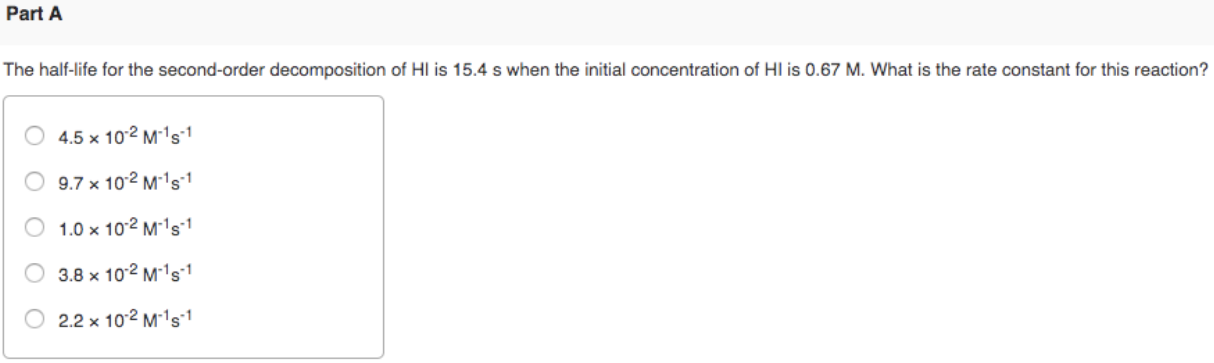# The half-life for the second-order decomposition of HI is 15.4 s when the initial concentration of HI is 0.67 M. What is the rate constant for this reaction? a) 4.5 x 10^-2 M^-1 s^-1 b) 9.7 x 10^-2 M^-1 s^-1 c) 1.0 x 10^-2 M^-1 s^-1 d) 3.8 x 10^-2 M^-1 s^-1 e) 2.2 x 10^-2 M^-1 s^-1